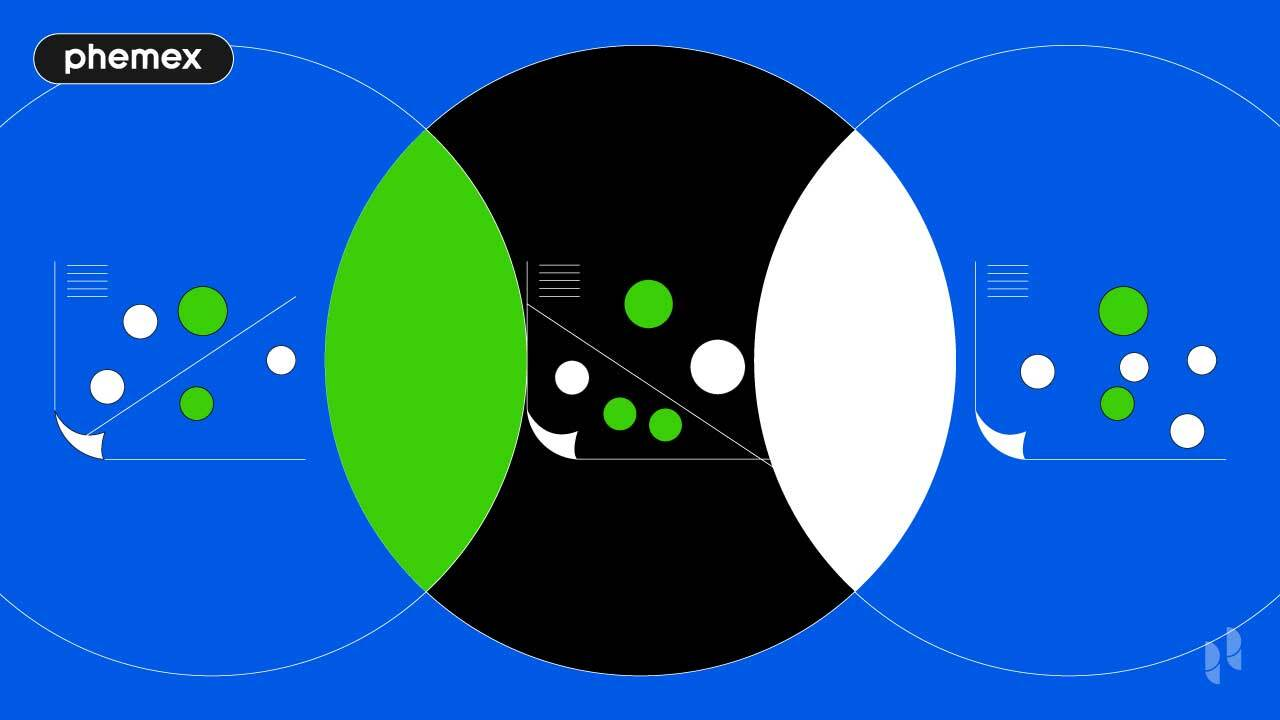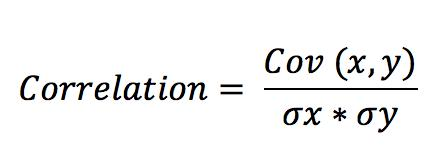Academy > Technical Analysis > What Is Correlation Coefficient: The Essential Diversification Tool >

# What Is Correlation Coefficient: The Essential Diversification Tool

2022-07-01 10:41:52

The upside of investing in cryptocurrency is evident, but the crypto market is notoriously volatile and risky compared to traditional asset classes like stocks and bonds. Timing the market is extremely difficult if not impossible.

Fortunately, there are ways to limit your exposure. The correlation coefficient is a key tool for any crypto investor serious about diversification.## What Is the Correlation Coefficient?

A correlation coefficient measures the strength of the relationship between the relative movements of two variables. It is a particularly useful tool in finance and investing, as it shows us the correlation between the price of two assets.

The correlation coefficient value will always range between –1.0 and 1.0.

Here is what the different values indicate:

• A negative value indicates an inverse relationship between the two assets—that is, when A decreases, B will increase, and vice versa. The perfect example of a negative correlation coefficient is the value of the US dollar versus the price of gold. The weaker the dollar, the higher the price of gold.
• A positive value indicates that the two assets move in the same direction. When asset A’s price increases, so does asset B’s. For example, when gold’s price increases, so do the gold mine’s profits and margins, thus increasing the stock price.
• A value of around zero means that the two assets have no relationship and move in completely unrelated ways.

The closer the number is to the extremes, the stronger the relationship. Correlation is only considered relevant if the coefficient is higher than 0.8 or lower than –0.8. So if two assets have a correlation coefficient of 0.3, then they can be considered as uncorrelated assets.## How to Find the Correlation Coefficient?

Finding the correlation coefficient is straightforward, using the CORREL function in Excel or Google Sheets. It takes two sets of data—for example, the price of Bitcoin and gold at the end of every month—and calculates the correlation between the two variables.

Alternatively, you can use an automatic asset correlation calculator such as Macroaxis or Cryptowatch.

## What Is the Correlation Coefficient Formula?

Most investors use automated tools to find the correlation coefficient, but here is a complete breakdown of the formula for those interested in the technical details.

For the sake of investing, the Pearson correlation formula proves to be the most useful. The first thing to determine is the two variables’ covariance, that is, the measurement of how they change together. Next, you find the standard deviation, meaning the dispersion of data from the average.

Finally, you divide the correlation coefficient by the standard deviation to get your correlation coefficient.Correlation coefficient formula

## How Does the Correlation Coefficient Apply to Investing?

Knowing the relationship between asset classes is very useful in investing. For starters, you can use correlation to determine how well your investment has performed compared to a benchmark index or another asset.

The correlation coefficient can also be used to maximize profit by identifying assets with strong positive correlations that grow together.

However, the correlation coefficient’s most useful application is the diversification benefit. Having a portfolio of negatively correlated assets reduces overall risk, or volatility, because  when part of your portfolio falls, the rest surges, minimizing capital gain losses.

The correlation coefficient also allows investors to determine when a correlation changes, proving a useful tool for deeper financial analysis. Let’s take the stock of a Bitcoin (BTC) mining company as an example. Profits tend to go up with the price of Bitcoin. So if the stock price is falling while the price of Bitcoin is rising, then there is likely an internal and fundamental issue within the company.

## What Are Some Correlation Coefficient Examples in Crypto?

Bitcoin is the undisputed crypto leader in terms of market cap. As such, the moves Bitcoin makes naturally impact the whole cryptocurrency market. This is mainly due to the fact that it is strongly or at least mildly correlated with most crypto assets.

Let’s look at how much cryptocurrencies correlated with Bitcoin last year:

CurrencyCorrelation coefficient
ETH0.78
LTC0.7
XRP0.64
USDT0.22
SOL0.32

BTC has the highest correlation with ETH, likely because both digital assets have seen massive institutional backing. Other crypto assets are less correlated, but Bitcoin still has an impact on their price action. The only exceptions are Solana (SOL) and Tether (USDT), which have a very weak correlation with BTC. Solana is an emerging project, and newer and smaller coins tend to be less correlated, while Tether is a US dollar stablecoin and not a decentralized finance (DeFi) asset.

However, the correlation differs greatly depending on the time period. For instance, in March of this year, the correlation coefficients were as follows:

CurrencyCorrelation coefficient
ETH0.88
LTC0.78
XRP0.59
USDT0.01
SOL0.63

Finding the precise cause of these changes isn’t always possible, as countless variables are in play. It often depends on what large investment funds are holding, selling and buying. As a general rule, ETH, LTC, ADA, SOL, BNB, and most other cryptocurrencies increase correlation with Bitcoin whenever there is a change in price to the upside, as was the case in March.

The main issue with relying solely on the correlation coefficient is that it changes drastically over time.

Generally, average correlation coefficient values are lower in the quarters when the market is on a downtrend, and higher when it is on an upward trend.

For example, BTC and ETH had a correlation coefficient of only 0.28 during Q2 of 2021 when Bitcoin’s price crashed by 43%, before rising to a correlation of 0.96 when Bitcoin reclaimed over 30% in price the next quarter. The unpredictable and volatile nature of crypto assets makes using the correlation coefficient at times unreliable.

## What Is the Correlation Between Bitcoin and Traditional Assets?

Unlike large cryptocurrencies, Bitcoin has shown very little correlation with most traditional asset classes such as gold and stock market indexes. In fact, Bitcoin has often been negatively correlated with a lot of them.

AssetCorrelation coefficient
Grayscale GBTC0.8
Gold–0.59
S&P 5000.36

### Correlation Between Grayscale GBTC and Bitcoin

Not surprisingly, the exception to the rule is the Grayscale Cryptocurrency Trust, which allows investors to invest directly in BTC by buying their shares. The trust has a high correlation of 0.8 due to the fact that most of its holdings are BTC.

### Correlation Between Gold and Bitcoin

Bitcoin is often called digital gold due to its store-of-value properties. However, gold has a weak-to-mild negative correlation to Bitcoin of –0.59, which is even lower than most other traditional assets. This is mainly due to the fact that gold is a relatively stable asset, while Bitcoin is the opposite.

### Correlation Between Stock and Bitcoin

In 2021, Bitcoin had a correlation of 0.36 with the S&P 500. The correlation between stocks and Bitcoin has been slightly increasing over the years, and is up 14 points from 2020 and 32 from 2019. Although the data shows some weak-correlation stocks, they are still a good option for diversification, since the number is not big enough to be relevant.

## What’s the Best Correlation Coefficient Trading Strategy?

The correlation coefficient helps traders to pursue diversification opportunities.

To minimize portfolio volatility and risk, pick assets that have no correlation or negative correlation to the rest. This way, when there’s a price drop in some of your assets, others are more likely to remain stable and in some cases even appreciate.

As a cryptocurrency investor, you can diversify by adding assets like gold or stocks to your portfolio. These are not correlated with crypto and hence will stabilize your portfolio when the cryptocurrency market dips or crashes.

For crypto-only portfolios, correlation coefficients may not be the most feasible tool as a long-term investment strategy. As the data shows, two cryptocurrencies can go from weak to strong correlation in a matter of months; it is thus more suitable for short-term investing or trading in crypto.## Conclusion

The correlation coefficient can prove very useful for cryptocurrency investors looking to hedge their portfolios. The data can help you pick the right assets to hedge with, minimizing the risks associated with investing. A well-hedged portfolio minimizes the effect of market crashes and provides the best risk-to-benefit equilibrium.

But be sure to do a full analysis and assess correlation during different periods. As good as the correlation coefficient may be, it is often unreliable by itself, particularly in crypto, so combine it with in-depth technical analysis for maximum accuracy.

Phemex | Break Through, Break FreeRegister to get \$180 Welcome Bonus!
Email